Conference Record of the 1998 IEEE International Symposium on Electrical Insulation, 7-10 June 1998, Washington, DC, USA. Vol. 2., pp. 607--610.

## Models of Streamers Growth with "Physical" Time and Fractal Characteristics of Streamer Structures

D. I. Karpov, A. L. Kupershtokh

Lavrentyev Institute of Hydrodynamics SB RAS
Novosibirsk, 630090, RUSSIA

### Abstract

The results of computer simulation of the streamer growth in three-dimensional space are presented. Various stochastic growth criteria and different methods of including the physical'' time into the models are discussed. All the models with physical'' time were tested. An implicit finite-difference method for solving the system of equations for the graph was developed. A great number of numerical experiments was carried out for different magnitude of applied voltage and conductivity of streamer branches. Fractal dimension of simulated discharge patterns was examined.

### Introduction

The streamer growth occurs owing to the formation of new conductive phase regions in dielectrics. Modern computer simulations of this phenomenon are based on the idea of space discretization. New linear segments of streamer channels join sequently neighbor sites of some spatial lattice to the streamer structure. The streamer shape is represented by a connected graph consisting of conductive bonds.

All growth criteria considered below are based on two main assumptions. Firstly, the growth is stochastic in time. Secondly, the probability of streamer growth is proportional to some function of local electric field r(E) depending on dielectric properties of a substance. Thus, the generation of new bonds is governed by some stochastic growth criteria in each time step.

The electric field potential was obtained by solving the Poisson equation. Charge transport along the streamer branches was computed according to Ohm's law for each conductive element.

### "Physical" Time and Growth Criteria

A sequence of time intervals for each growth step calculated in a proper way following certain assumptions can be named the "physical" time. Apparently, there are only two ways to introduce the "physical" time into stochastic models of streamer growth at electric breakdown. One of them is that the time step t is taken at first. Then, all bonds that have time to arise according to the some stochastic criteria are accepted. Another way of looking at it is to consider the delay time of appearance of the first new bond as the "physical" time interval of this growth step. Based on such classification, all the models can be divided into two groups. First group consists of models in which only one new bond is added in a time step (single-element models). The models of second group allow generation of several bonds per time step and can be named multi-element models.

In single-element models, we actually have to take both the new bond and the "physical" time interval in a stochastic way.

#### Niemeyer--Pietronero--Wiesmann Model

In the model proposed by Niemeyer, Pietronero, and Wiesmann (NPW) in 1984 , the probability of streamer growth was related to local electric field. In each step of growth procedure, one of the candidate lattice sites i was added to the streamer structure according to following probability distribution

p(Ei ) = r(Ei ) / $\sum _{i=1}^n$ r(Ei ).

For the first time this model allowed one to simulate the formation of branching streamer structures having fractal dimension.

#### Field Fluctuation Model

The field fluctuation criterion (FFC) proposed in [2-4] allows the generation of several new conductive bonds per each time step t. At each lattice site where the local field

Ei > E* - di ,             (1)

a new region of a conductive phase arises. A random value d is assumed to take into account inhomogeneities in dielectrics, thermal and other fluctuations, including local microfields acting on the molecules.

The following probability distribution for fluctuations d is used

j(d) = exp(-d / g) / g ,             (2)

that is d = - g ln(x). Hereinafter x will be a random number that is uniformly distributed in the interval from 0 to 1. For a given distribution function, r(E) has the form

r(E) = A eE/g ,             (3)

where

A = $\frac${1}{t} exp(-E* / g) ,

where A and g are constants that depend on dielectric properties of liquid. The quantity of E* should be always chosen greater then E. After this, t should be accordingly changed to ensure A=const.

To each random value di corresponds the time of appearance of the i-th bond

ti = - $\frac${ln(1-exp(-di / g))} {r(Ei)}.             (4)

The distribution of times ti is

j (ti ) = r(Ei ) exp(-r(Ei ) ti ).             (5)

Thus, condition (1) is exactly equivalent to the inequality ti < t.

#### Biller's Model

In 1993 Biller  proposed a growth criterion based on the idea of introducing the delay time of generation of each candidate bond, ti= - ln(xi ) / r(Ei ), by analogy with the statistical time lag of breakdown. In the step of growth, only one bond that has the shortest delay time was added to the streamer structure. Precisely this quantity t = min\limits_{i}\{ti\} was takenas "physical" time interval.

#### Scaled Stochastic Time Models

Distribution function(5)of new bond generation in time t is the same for both the FFC and Biller's models. The probability of the event that the new bond between streamer structure and some lattice site i does not arise in time t , is equal to

p(Ei ,t) = $\int$\limits_{t}^{$\infty$} r(Ei ) exp(-r(Ei ) t) dt = exp(-r(Ei ) t).

Let there be n dielectric sites near the structure. Then the probability that no bonds arise in time t is equal to

P(t) = $\prod$\limits_{i=1}^{n} p(Ei ,t) = exp(-Snt),

where Sn = Si=1n r(Ei ) . The corresponding distribution function of the time of appearance of the first new bond is f(t) = Snexp(-Snt) . It has been proposed  to use the random value tS = - ln(x) / Si=1n r(Ei ) --- the "scaled stochastic time" --- as the "physical" time interval.

At the same time, a new bond in the single-element model can be selected in several ways:

• for instance, by adding, as in , the bond that has minimum delay time
ti = - ln(xi ) / r(Ei ) to the conductive structure (see in );
• using the NPW criterion;
• using the FFC. A bond that has the shortest of the generation times ti calculated from Eq. (4) is chosen.
All these models can be named Scaled Stochastic Time Models (SSTM).

#### New Multi-Element Models

In the present work, other two models with "physical" time were proposed within the framework of the definition of multi-element models.

• The times ti = - ln(xi ) / r(Ei ) is computed for all candidate bonds as in Biller's model. All the bonds having ti < t arise.
• Several time steps tSj = -ln(xj ) / Si=1n r(Ei ) are sequentially performed as in SSTM as long as Sj=1m tSj < t.

### Discussion

In , the choice of the new bond was performed according to the NPW criterion but the time interval was calculated from the formula t* = 1 / Si=1n r(Ei ) . This expression is the mean value of the scaled stochastic time step  < tS . Although the choice of the bond was stochastic as in the NPW model, the time was deterministic. In fact, the temporal scale is not uniform in these models. For example, the natural course of time is broken for prebreakdown current. Therefore, the main shortcoming of both these models is that the "physical" time and, consequently, the statistical time lag are really absent in them.

The remaining growth criteria with the "physical" time that were considered above (FFC, SSTM, Biller's, and modifications of them) use the same distribution (5) of the stochastic time and, therefore, describe correctly, in particular, the statistical time lag of breakdown. Thus, these models are essentially equivalent, provided that the function r(E) = A e-E/g is the same.

The basic difference of single-element models from multi-element models is that they consider the competition between candidate bonds in different ways. The competition is neglected in multi-element models, and the first bond that appears is assumed to suppress the growth of other bonds in single-element models. All multi-element models allow one to introduce a constant time interval for all steps of the growth. This fact is important for gas-dynamics flow simulation.

The time step is decreased because of the competition among the great number of branches during simulation of the streamer propagation by single-element models. It is reasonable to continue the computations using one of the multi-element models in this case.

The FFC criterion of conductive phase generation satisfies a specific form (3) of the function r(E), which is considered to describe dissociation and ionization in a strong electric field. All other models allow us to use any other dependences r(E) corresponding to various physical mechanisms of streamer inception and propagation. For example, one can use the well-known dependence r(E) = (E/E0 )h / t0 or the tunnel effect r(E) = exp(-C/E ) / t0 .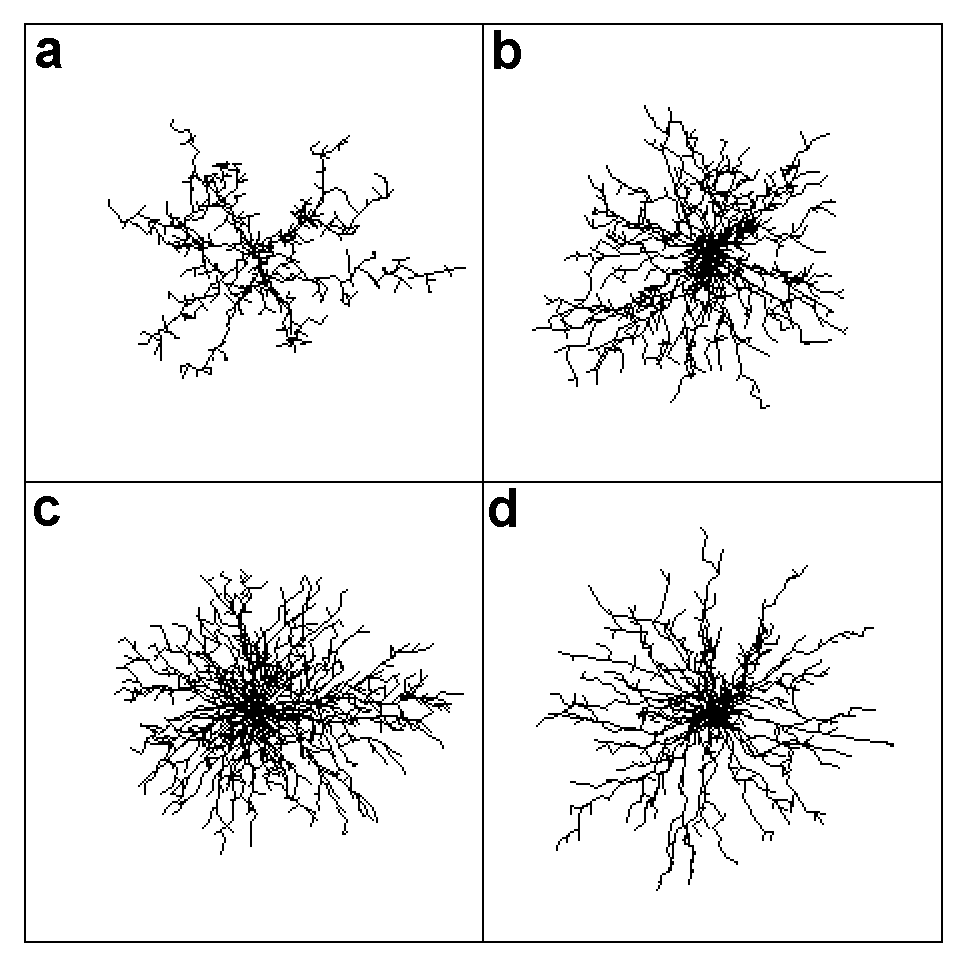Figure 1. Patterns of streamer structures obtained with FFC. s = 1: V = 5(a), 10(b), and 15(c) ; s = 10: V = 15(d).Figure 2. Patterns of streamer structures obtained with Biller's model. s = 1: V = 5(a), 10(b), and 15(c) ; s = 10: V = 15(d).

### Computer Simulation

Computer simulation of streamer growth was carried out in three dimensions. M = 26 permissible directions (including diagonals) of channel propagation was used at each site of a cubic lattice to diminish the anisotropy of the growing structure. Thus, the charges flow according to Ohm's law along each bond of a connected graph forming the frame of the conductive structure. The charge transport and the electric field potential distribution are governed by the continuity and Poisson equations

L(j) = - 4 p Q/h3 ,

$\frac{dQ}{dt}$ = - $\Div$(Im) ,

Im = - sm s   D jm / lm ,

where L is the finite-difference Laplace operator, h is the lattice bond length, $\Div$(Im ) = Sm=1M Im is the sum over all currents Im flowing out of some vertex of the graph, M is the number of neighboring graph vertices, Djm is the electric potential difference along the bond, lm is the bond length, s is its cross section, and sm is its conductivity.

We used time-implicit finite-difference method for the equation of charge transport to obtain the equation for computation of electric potential

L(jn+1)h3 + 4 p t s   \Div(sm   Djmn+1 / lm) = - 4 p Qni,j,k ,

where t is a step in time and n is its number. Charge transport is computed using new values of the electric-field potential jn+1

Qn+1i,j,k - Qni,j,k = s t Sm=1M sm Djmn+1 / lm

to ensure charge conservation.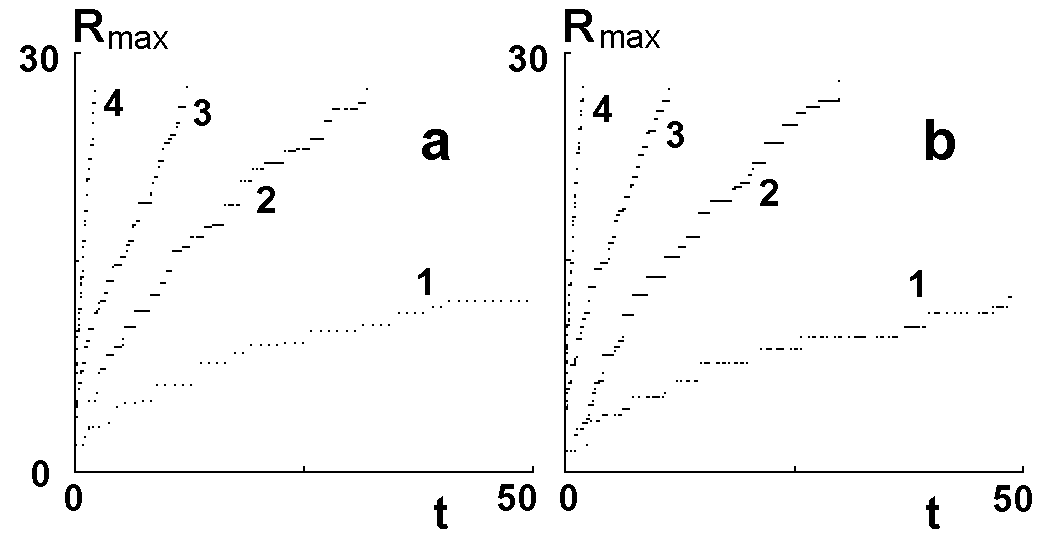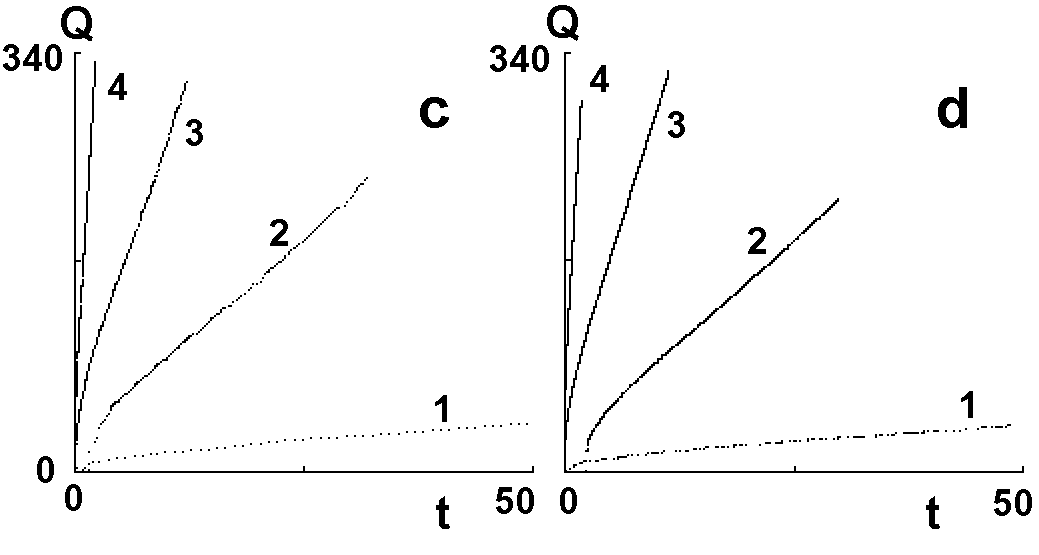Figure 3. FFC (a and c) and Biller's (b and d) models. Curves 1, 2, and 3 refer to V = 5, 10, and 15 (s = 1); curve 4 refers to V = 15 (s = 10).

The calculation method developed is stable over a wide range of parameter 4 p s t s / h2 up to 105.

The simulation was carried out on lattices with sizes up to 90x90x90. The conductivity s was assumed to be constant and equal for all branches of the streamer structure. Streamer propagation velocity was ensured equal for all bonds including both types of diagonals, provided that the electric potential difference between their ends was the same. This statement was tested specially.

### Results

It is more convenient to investigate and compare geometric characteristics of streamer structures by simulation of electric breakdown in a spherically symmetric field.

For example, typical streamer structures obtained with multi-element FFC model (Fig. 1) and single-element Biller's model (Fig. 2) are shown. A denser streamer structure is formed when the applied voltage V increases, provided the conductivity is the same (Figs. 1,a--c and 2,a--c). Increase in s is equivalent to reduction of V (Figs. 1d and 2d). There is an area that is filled densely with streamer channels in the vicinity of the central electrode. These patterns coincide within stochastic variations. The time dependences of the growth velocity (Fig. 3a,b) and the total charge of structure (Fig. 3c,d) practically coincide for all the models with "physical" time provided that the function r(E) is the same. An increase in s causes a strong rise of the growth velocity (curves 4 in Fig. 3a,b) owing to the reduction of the charge relaxation time in the channels. The maximum electric field strength in front of the streamer tips is roughly constant during the growth and depends mainly on V and s .

Figures 4 and 5 show the graphs of the logarithm of the conductive bond density averaged over 15 streamer patterns versus the logarithm of the distance r from the centre. There is no fixed value of the fractal dimension for the streamer structure at finite s . The differential fractal dimension Dr introduced in  depends on r . Dr is close to the space dimension near the central electrode and reduces when the radius increases, particularly in the growth region. The area with
Dr » 3 expands as voltage increases (Fig. 4a,b). The electric field there is strong so that the streamer channels can propagate rapidly without charge relaxation in them.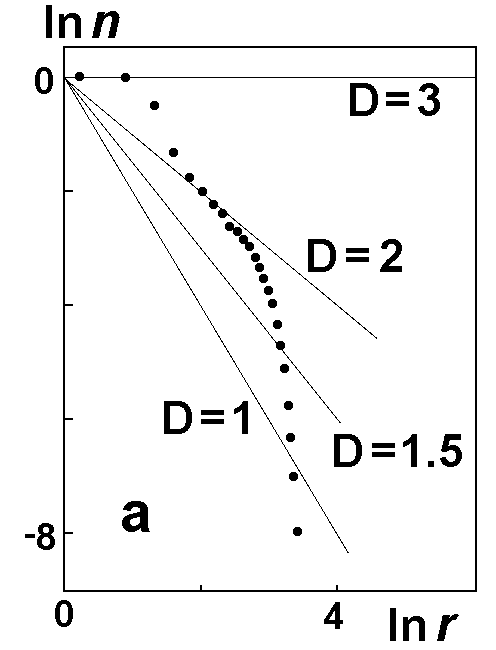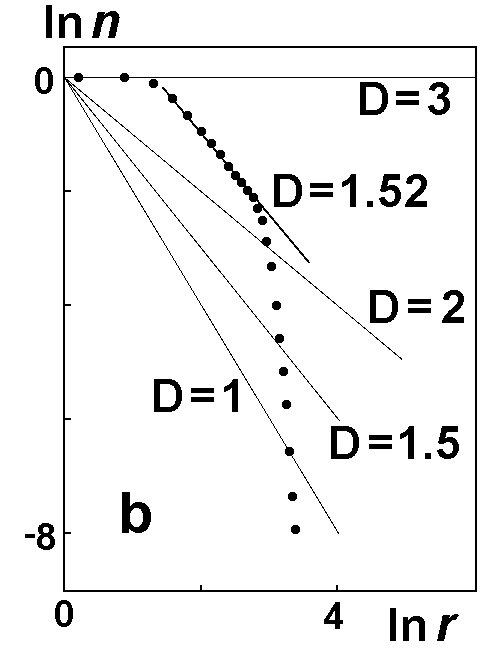Figure 4. Differential fractal dimensions of streamer structures for FFC model. V = 10(a) and 30(b) (s = 1).

Some discrepancies between single-element and multi-element models exist in the high-field region in the vicinity of the central electrode. There multi-element models give a higher density of streamer channels (Fig. 5b). These differences practically vanish in the case where the time step t in multi-element models is small, and, hence, the competition of neighboring bonds becomes insignificant.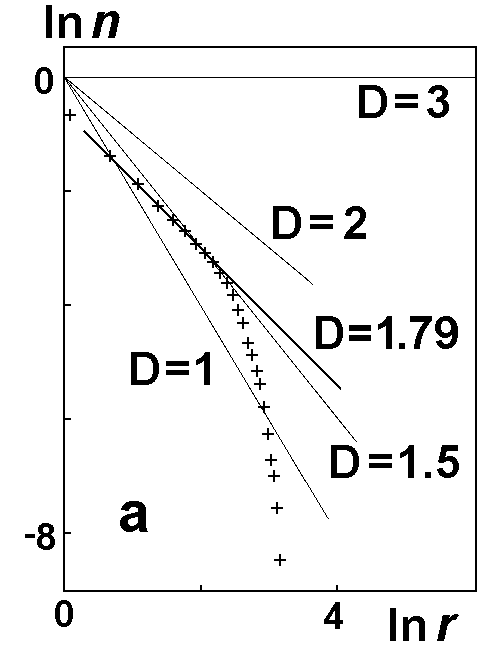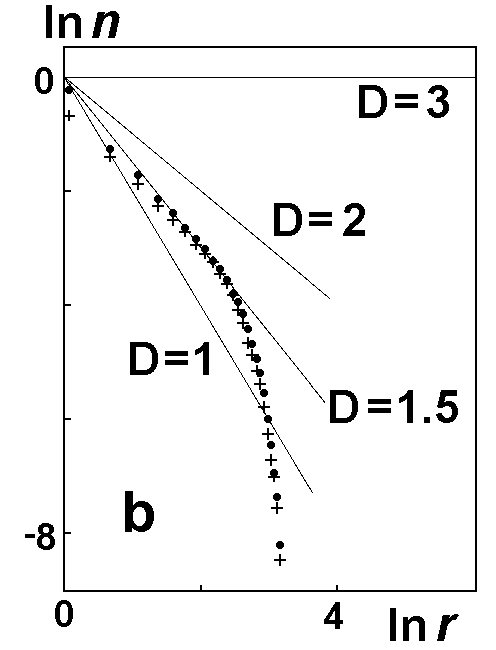Figure 5. Differential fractal dimensions of streamer structures. (a) Biller`s model, (b) FFC (·) and Biller's (+) models, V = 5, s = 1.

The same simulations were performed with SST models and new multi-element modification of Biller's model. These results agree with those described above.

### Conclusion

The comparison of differential fractal dimensions obtained with the growth criteria discussed above shows a little discrepancy between single-element and multi-element models. Except for this circumstance, all the models with "physical" time are essentially equivalent. All of them describe correctly the propagation velocity of streamer tips, incomplete discharges, and the distribution of the statistical time lag. The simulation results are qualitatively similar to the well-known experimental data on nanosecond breakdown in dielectric liquids.

### Acknowledgements

This work was supported by Russian Foundation for Basic Research under grant No. 97-02-18416.

### References

1. L. Niemeyer, L. Pietronero, H. J. Wiesmann, "Fractal dimension of dielectric breakdown," Phys. Rev. Lett., Vol. 52, pp. 1033--1036, 1984.

2. A. L. Kupershtokh, "Simulation of Fractal Structure of Electrical Breakdown in Condensed Dielectric," in: Abstracts of reports of 5th All--Union Workshop: Physics of pulse discharges in condensed media, Nikolaev, USSR, pp. 56--57, 1991. (in Russian).

3. A. L. Kupershtokh, "Fluctuation model of the breakdown of liquid dielectrics," Sov. Tech. Phys. Lett., Vol. 18, No. 10, pp. 647--649, 1992.

4. A. P. Ershov, A. L. Kupershtokh, "Fluctuation model of liquid dielectric breakdown with incomplete charge relaxation," in: Proc. of the 11th Int. Conf. on Conduction and Breakdown in Dielectric Liquids, IEEE No. 93CH3204--5, Baden-Dättwil, Switzerland, pp. 194--198, 1993.

5. P. Biller, "Fractal streamer models with physical time," in: Proc. of the 11th Int. Conf. on Conduction and Breakdown in Dielectric Liquids, IEEE No. 93CH3204--5, Baden-Dättwil, Switzerland, pp. 199--203, 1993.

6. A. L. Kupershtokh, "Propagation of Streamer Top between Electrodes for Fluctuation Model of Liquid Dielectric Breakdown," in: Proc. of the 12th Int. Conf. on Conduction and Breakdown in Dielectric Liquids, IEEE No.96CH35981, Roma, Italy, pp. 210--213, 1996.

7. V. V. Lopatin, M. D. Noskov, V. R. Kukhta, "Fractal description of discharge propagation in liquid," in: Proc. of the 11th Int. Conf. on Conduction and Breakdown in Dielectric Liquids, IEEE No. 93CH3204--5, Baden-Dättwil, Switzerland, pp. 204--208, 1993.

Back to Laboratory Home Page or to A.L.Kupershtokh